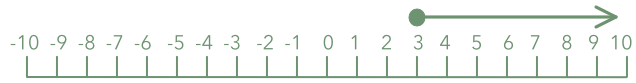Solving Linear Inequalities

# Solving Linear Inequalities

GCSE(F), GCSE(H),

Solving an inequality means determining the values for which an inequality is true. Much of the process is similar to solving an equation: the solution is found by applying the same operations to both sides of the inequality to simplify the inequality.

Once the inequality has been simplified, select values to test in the original inequality to make sure that the solution is true.

One difference between solving equations and solving inequalities is when multiplying the inequality by a negative number. In this instance, as well as changing the signs of the terms, the inequality sign also has to be reversed.

An inequality that describes a range are solved in the same way: operations are applied to all three parts of the inequality until a single value of the variable is left.

## Examples

1. Solve 6 - 4x ≥ 6(2 - x) and show on a number line.

Answer:6 - 4x ≥ 6(2 - x)

6 - 4x ≥ 12 - 6x (multiplied out the brackets)

-4x ≥ 6 - 6x (subtracted 6 from both sides)

2x ≥ 6 (added 6x to both sides)

x ≥ 3 (divided both sides by 2)

Check: 6 - 4(3) ≥ 6(2 - 3) which is -6 ≥ -6 (true). Check also for a true value; put x=5: 6 - 4(5) ≥ 6(2 - 5) which is -14 ≥ -18 (also true).

2. What integers satisfy 9 < 2x + 3 < 19?

Answer: 4, 5, 6 and 7

9 < 2x + 3 < 19

6 < 2x < 16 (subtracted 3 from each part)

3 < x < 8 (divided each part by 2)

The integers that satisfy the inequality are greater than 3 and less than 8.

Check: check the left hand part: 9 < 2(3) + 3 = 9. Check the right hand part: 2(8) + 3 = 19. Check a value from the answer: 9 < 2(5) + 3 < 19 which is 9 < 13 < 19 (true).